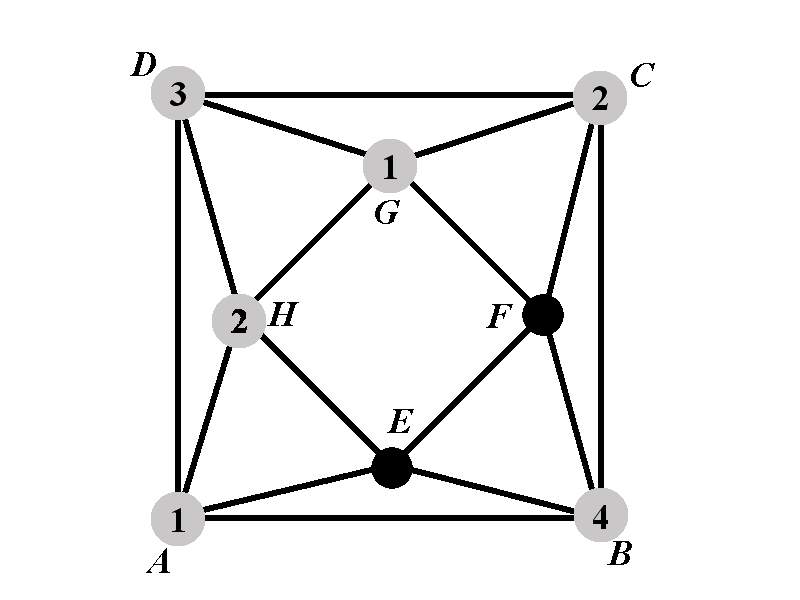Back to: Chromatic Polynomial Case $$2.2.1^{\circ}$$
##### Case 2.2.1.1: Calculation of $$P_{2.2.1.1^{\circ}}(k)$$We need to determine the number of ways to assign colors to the vertices $$E$$ and $$F$$. Notice that none of the vertices $$E$$ and $$F$$ can have any of the colors $$1$$, $$2$$, and $$4$$. Also, the vertices $$E$$ and $$F$$ must have distinct colors. It remains to notice that there are no other restrictions on the colors of $$E$$ and $$F$$. Therefore the number of ways to assign a color to $$E$$ is $$k-3$$. For each assignment of color to $$E$$ there are $$n-4$$ possible colors to assign to $$F$$. Therefore $P_{2.2.1.1^{\circ}}=(k-3)(k-4).$